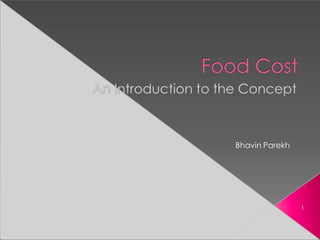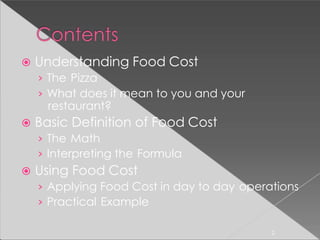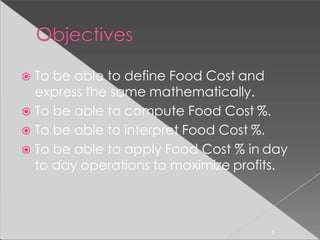Successfully reported this slideshow.

# FOOD COSTING.pptx×

# FOOD COSTING.pptx

ONE OF THE MOST INTRESTING SESSIONS IN FOOD PRODUCTIONS. IDEAL FOR ALL THE STUDENTS TO GO THROUGH IT.

ONE OF THE MOST INTRESTING SESSIONS IN FOOD PRODUCTIONS. IDEAL FOR ALL THE STUDENTS TO GO THROUGH IT.

### FOOD COSTING.pptx

1. 1. Bhavin Parekh 1
2. 2.  Understanding Food Cost › The Pizza › What does it mean to you and your restaurant?  Basic Definition of Food Cost › The Math › Interpreting the Formula  Using Food Cost › Applying Food Cost in day to day operations › Practical Example 2
3. 3.  To be able to define Food Cost and express the same mathematically.  To be able to compute Food Cost %.  To be able to interpret Food Cost %.  To be able to apply Food Cost % in day to day operations to maximize profits. 3
4. 4. 4
5. 5. Pizza 1 person gets the whole pizza i.e. 1 person = 100% 2 persons get half a pizza each. i.e. 2 person = 50% 4 persons get only a quarter pizza each. i.e. 4 person = 25% 50% 5 50% 25% 25% 25% 25%
6. 6. Rs. 300 Rs. 1000 6 Assume that your total sale for the day is Rs. 1000/- Your product is food. In order to produce the food to sell, you incurred a cost of Rs. 300. Your net saving at the end of the day will be Rs. 700 (Rs. 1000 – Rs. 300). This can also be referred to as PROFIT. In order to increase your profit, you will have to reduce the food cost, given that the total sale is a constant.
7. 7. Food Cost is defined as the cost incurred to produce the food to be sold. This is expressed in percentage (%). Hence, Food Cost % is mathematically defined as the ratio of the cost of food to the total sales. It is mathematically expressed as: Food Cost % = Cost of Food x 100 Total Sale 7
8. 8.  The food cost is directly proportional to the cost of food. › This implies that the higher the cost of ingredients, the higher will be the Food Cost %. › The higher the Food Cost %, the lower is your profit. 8
9. 9.  The food cost is indirectly proportional to the total sales. › This implies that higher the sale, the lower is the cost of Food Cost % and vice versa.  What is the impact of Food Cost % on your restaurant’s profit ? 9
10. 10. Pizza The higher the cost of ingredients used to prepare the dish, the higher is the Food Cost %. The higher the total sale, the lower is the Food Cost % and vice versa. A higher Food Cost % will eat into a larger share of your pizza, leaving you with a smaller piece and vice – versa. 10
11. 11.  The formula.  The independent and dependent variables.  The impact of Food Cost % on profit. 11
12. 12. Objective Pricing Methods  If you want to keep your Food Cost as 40% then:  Multiplier = 1 / 40% = 1 / .40 = 2.5 Assume that a Seafood Platter has a Standard Food Cost . Portion of a Seafood Platter is \$ 5.32  If a Food Cost % of 40% is desired:  Base Selling Price (B.S.P.) = \$ 5.32 x 2.5 = \$ 13.30
13. 13. Assume that a Seafood Platter has a Standard Food Cost / Portion of a Seafood Platter is \$ 5.32  If a Food Cost % of 40% is desired:  Base Selling Price (B.S.P.) = \$ 5.32 x 2.5 = \$ 13.30
14. 14. 14